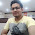### How to check whether a string is numeric or not in Asp.net C#,VBIntroduction: In this article i am going to share the code trick to check whether entered string is numeric/number or not in asp.net using both C# and VB languages.

Description: While working on asp.net project i got the requirement to test whether the entered string is number or not? The solution is very easy and i have created a function for this purpose and  i am going to share the solution with all so that developers can implement it in their project easily whenever required.

Implementation: Let's create a demo small web application to test the string is number or not.

Asp.Net C# Section
• In the <Form> tag of the design page(default.aspx) design the page as:

<div>
<fieldset style="width:200px;">
<legend>Check String is numeric or not</legend>
<center>
<table>
<tr>
<td>
<asp:TextBox ID="txtTest" runat="server"></asp:TextBox>
</td>
</tr>
<tr>
<td>
<asp:Button ID="btnTest" runat="server" onclick="btnTest_Click"
Text="Test String" /><br />
<asp:Label ID="lblStatus" runat="server"></asp:Label>
</td>
</tr>
</table>
</center>
</fieldset>
</div>

• In the code behind file(default.aspx.cs) write the code as:

protected void btnTest_Click(object sender, EventArgs e)
{
if (IsNumeric(txtTest.Text.Trim()))
{
lblStatus.Text = txtTest.Text + " is Numeric";
lblStatus.ForeColor = System.Drawing.Color.Green;
}
else
{
lblStatus.Text = txtTest.Text + " is not Numeric";
lblStatus.ForeColor = System.Drawing.Color.Green;
}
}

public bool IsNumeric(String str)
{
try
{
if (!string.IsNullOrEmpty(str))
{
int num;
if (int.TryParse(str, out num))
{
return true;
}
}
}
catch (Exception ex)
{
lblStatus.Text = "Error occured : " + ex.Message.ToString();
lblStatus.ForeColor = System.Drawing.Color.Red;
}
return false;
}

Note: Above created function "IsNumeric" consider only integer numbers e.g. 123 to be numeric. But if you want above function also consider the string like 123.5 i.e. the values with decimals to be numeric then you can replace the following two lines
int num;
if (int.TryParse(str, out num))

with these two lines:

double num;
if (double.TryParse(str, out num))

Now it will also consider the string with decimals e.g. 45.8 etc to be numeric.

Asp.Net VB section:
• In the <Form> tag of the design page(default.aspx) design the page as:

<div>
<fieldset style="width:200px;">
<legend>Check String is numeric or not</legend>
<center>
<table>
<tr>
<td>
<asp:TextBox ID="txtTest" runat="server"></asp:TextBox>
</td>
</tr>
<tr>
<td>
<asp:Button ID="btnTest" runat="server" Text="Test String" /><br />
<asp:Label ID="lblStatus" runat="server"></asp:Label>
</td>
</tr>
</table>
</center>
</fieldset>
</div>

• In the code behind file (default.aspx.vb) write the code as:

Protected Sub btnTest_Click(sender As Object, e As System.EventArgs) Handles btnTest.Click
If IsNumeric(txtTest.Text.Trim()) Then
lblStatus.Text = txtTest.Text + " is Numeric"
lblStatus.ForeColor = System.Drawing.Color.Green
Else
lblStatus.Text = txtTest.Text + " is not Numeric"
lblStatus.ForeColor = System.Drawing.Color.Green
End If
End Sub

Public Function IsNumeric(str As [String]) As Boolean
Try
If Not String.IsNullOrEmpty(str) Then
Dim num As Integer
If Integer.TryParse(str, num) Then
Return True
End If
End If
Catch ex As Exception
lblStatus.Text = "Error occured : " & ex.Message.ToString()
lblStatus.ForeColor = System.Drawing.Color.Red
End Try
Return False
End Function

Note: Above created function "IsNumeric" consider only integer numbers e.g. 123 to be numeric. But if you want above function also consider the string like 123.5 i.e. the values with decimals to be numeric then you can replace the following two lines
Dim num As Integer
If Integer.TryParse(str, num) Then

with these two lines:

Dim num As Double
If Double.TryParse(str, num) Then

Now it will also consider the string with decimals e.g. 45.8 etc to be numeric.

Now over to you:
" I hope you have got How to Check whether entered string is numeric or not in Asp.Net and If you like my work; you can appreciate by leaving your comments, hitting Facebook like button, following on Google+, Twitter, Linked in and Pinterest, stumbling my posts on stumble upon and subscribing for receiving free updates directly to your inbox . Stay tuned and stay connected for more technical updates."
Previous
Next Post »

Anonymous

Does your function consider 3.14 to be numeric?